# Fourier's Law

New for April 2016! ;On this page we will discuss transient heat flow, according to Fourier's Law, then show you an example of numerical integration using Excel. Joseph Fourier is credited with Fourier's Law, which we discuss on this page. You can find Fourier in the Microwave Hall of Fame!

Fourier's Law can be fully described in the partial differential equation for heat conduction in solids: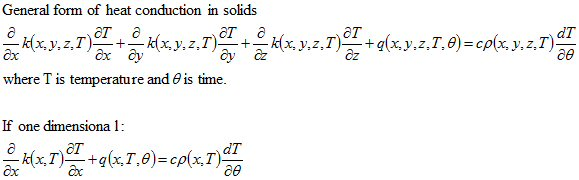Sorry about the gnarly equation graphics, we'll do something about that in the future.

After more than one hundred years, not many useful closed-form solutions exist for this partial differential equation, for non-steady-state problems. But enter cheap numerical analysis in the second half of the 20th century, and now any transient problem can be solved. Chances are you need some very expensive software, unless you really dumb down the problem.

## Numerical integration technique of one-dimensional transient heat flow using energy balance

Let's look at a one-dimensional heat transient, to make a simple analysis case.  This is similar to when you put an eight-inch cylinder of some material on top on a stove, and turn up the heat.  The bottom heats up first, and the heat spreads toward the top.  The temperature on the top is less than the temperature on the bottom, as long as the heat is applied.  The top surface can be adiabatic (nothing in the pot) or isothermal (when you are boiling water at sea level, the temperature will be stuck at 100C).

The basic premise is that time and distance “dimensions” can be treated independently so long as second law of thermodynamics is not broken:

Net energy into system must equal change in energy stored in system.

This numerical analysis technique dates to the 1930s, the reference used was Numerical Analysis of Heat Flow by G. M. Dusinberre, McGraw-Hill Book Company, 1949.

• Time is divided into intervals dθ
• Length is divided into small segments dx
• Present temperatures of element reference points are T1, T2, T3…
• Future temperatures are T1´, T2´, T3´.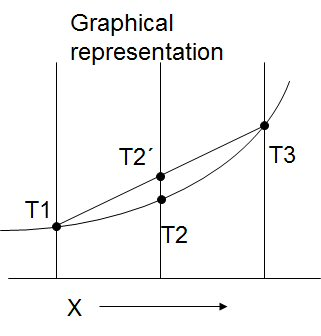An iterative procedure is used to calculate all reference points at successive time intervals… some assumptions:

• Only the differences between local temperature and temperature of adjacent reference points T1 and T3 need be considered in calculating the change of local temperature T2´.
• The change of heat storage in element T2 can be calculated from change in its reference point temperature.
• Criteria “M>2” must be obeyed.

Below is the coordinate system that is generally used in on-dimensional heat transient analysis.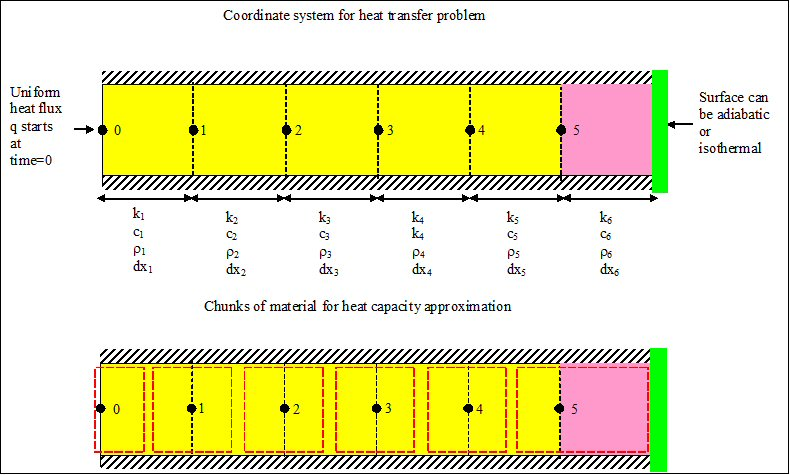If there is no internal heat, the equation reduces to: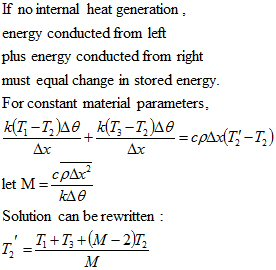Time and distance steps in heat flow are inexorably linked by that pesky differential equation. The dimensionless quantity M defines the relationship. Larger M means smaller time increments or longer distance increments. M defines how much the future temperature of a point depends on its present temperature. If M=2, present temperature is ignored, which can lead to choppy results in the first few time increments. The larger M is, the more dependence on present temperature. If M<2, the present temperature effect changes sign and calculation blows up! There is an important point about M that you need to understand:

When you analyze the one dimensional problem, you don't get to pick the distance increments, you only get to pick M. By trial and error, you can slice the material into desired increments. Smaller M makes smaller distance steps.

There are three cases to consider in the analysis. The first cell equations are: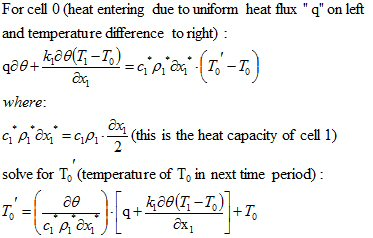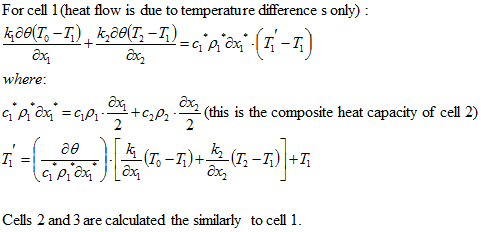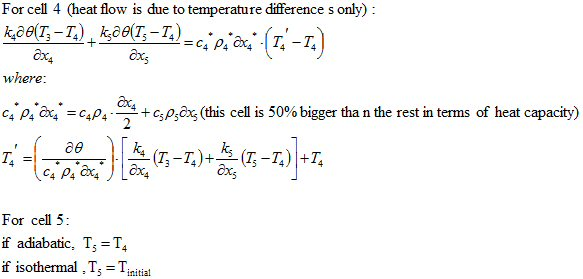## The Excel file...

If you think about those d/dx terms as small increments rather than a challenge for a math whiz to try to integrate (good luck with that) we now have the means to numerically solve for temperatures along a stack of materials. Only a fool would try to do that in Excel.... but one fool did! The Unknown Editor's transient thermal analysis tool was used to look at a stack of different materials, with a uniform heat flux (or even a non-uniform heat flux).

What else would we want our analysis tool be able to do? How about have a lookup table for material properties, and the ability to have four different materials in the "stack"? When we examine heterogeneous material stacks, Kleft does not necessary equal Kright…, nor are c and rho constants. At one boundary there is a heat source and no convection, nut heat flux is not necessarily constant, so we added means to change the heat flux with time. The second boundary can be either adiabatic or isothermal, you can choose.

Below is a view of the control panel in the Excel file.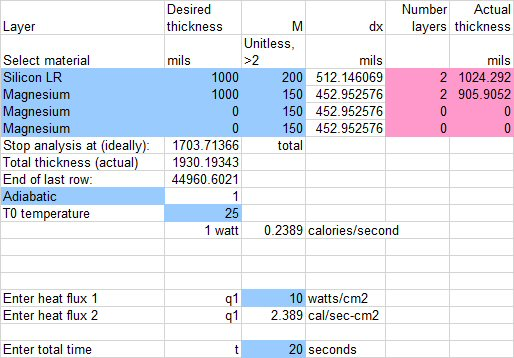The spreadsheet has 96 distance steps and 2000 time steps, or almost 100,000 cells. In the old days it would bring a computer to its knees when you changed a variable. Now it's almost instantaneous, however, it still measures 10MB. We will offer a zipped version of it in the download area, soon.

Below is the transient response of 1mm of silicon attached to 1mm of magnesium, with the heat injected on the silicon side and the other side adiabatic. When the heat starts, the stack is at 25C. The top surface (blue line) starts to heat up immediately, while the bottom surface (black line) has a bit of a time lag. Eventually, a fixed gradient is established between the top and bottom, about 17 degrees apart. More of the gradient is in the silicon, as it has lower conductivity.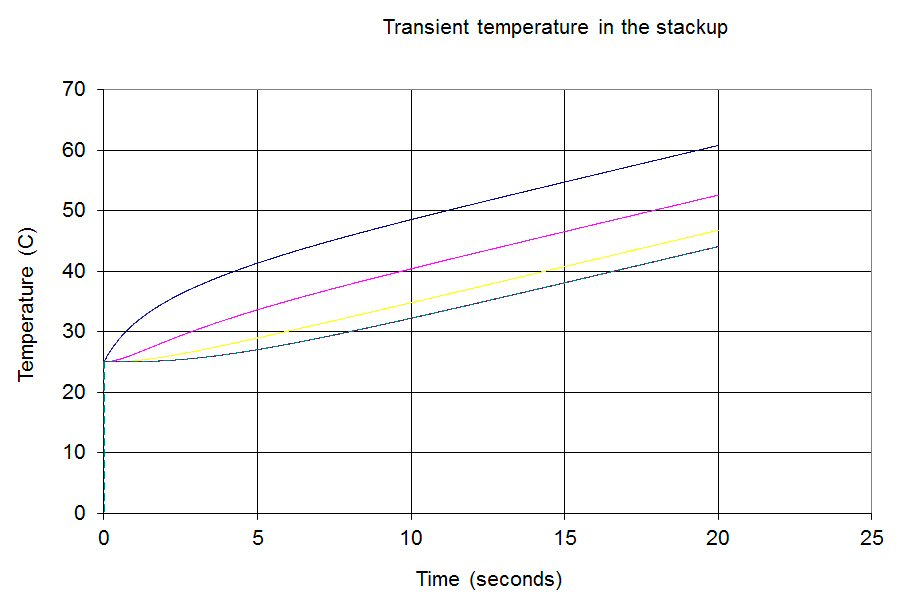If you don't think transient heat flow is interesting, consider another career besides electronics. You can make some serious money with a Kettle Corn franchise, and never be more than 5 feet from a tasty but nutrition-less snack. When you want to know how fast your kettle heats up, come back and visit.

Excel file is located here

More to come....

Author : Unknown Editor